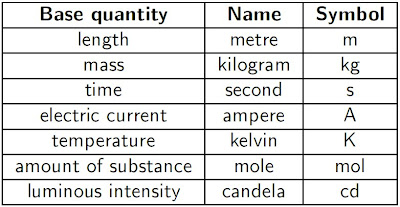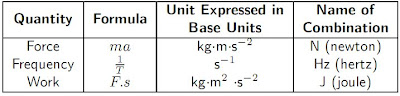## Saturday, November 21, 2009

### Understanding base quantities and derived quantities

Physical quantities are quantities that can be measured. They can be classified into:
(i)
Base quantities
A base quantities is a quantity that cannot be expressed in terms of other quantities.(ii) Derived quantities

A derived quantity is a quantity that is a combination of base quantities by meant of multiplication, division or both.

To make working with units easier, some derived units are given special names.It is very important that you are able to recognise the units correctly. For instance, the new- ton (N) is another name for the kilogram metre per second squared (, while the kilogram metre squared per second squared ( is called the joule (J).

Lesson review :

1. Explain what base quantities and derived quantities are.
2. list 5 base quantities and their units
3. list 5 derived quantities and their units.